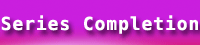# Series Completion Psychometric Test Papers | Verbal Reasoning## Series Completion Practice Paper

In each of the following series some of the letter(s) are missing which are given in that order as one of the alternatives below it. Choose the correct alternative.

1. T, R, P, N, L, ?, ?
(a) J, G
(b) J, H
(c) K, H
(d) K, I

2. CDE, UK, NOP, ?
(a) FGH
(b) RST
(c) TUV
(d) QRS

3. Y, S, U, O, Q, ?, ?
(a) NO
(b) LM
(c) JK
(d) HI

4. EAC, GCE, IEG, ?
(a) JHI
(b) KGI
(c) JGI
(d) KU

5. FLU, GMV, HNW, IOX, ?
(a) JYP
(b) WYP
(c) WPY
(d) JPY

6. A, P, C, Q, E, R, G, ?, ?
(a) S I
(b) H I
(c) I S
(d) T J

7. J, Q, I, R, H, S, ?, ?
(a) F T
(b) G T
(c) H T
(d) K T

8. CFL,EIK, GJ, IOI, ?
(a) KRH
(b) KRJ
(c) JRH
(d) KQH

9. A, Z, B, Y, C, X, ?, ?
(a) EV
(b) WD
(c) DW
(d) YV

10. AZY, EXW, IVU, ?
(a) MTS
(b) MQR
(c) NRQ
(d) LST

11. B, C, F, H, L, ?, ?
(a) XY
(b) MN
(c) QW
(d) OP